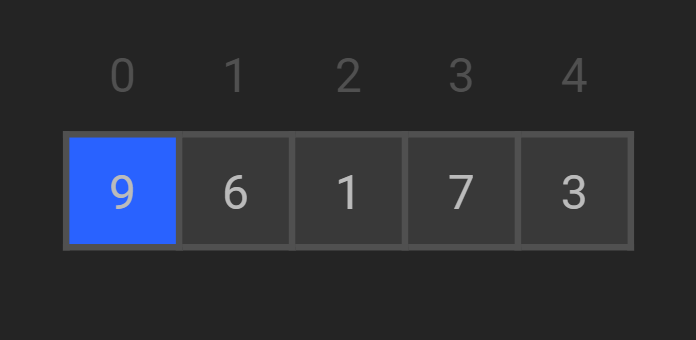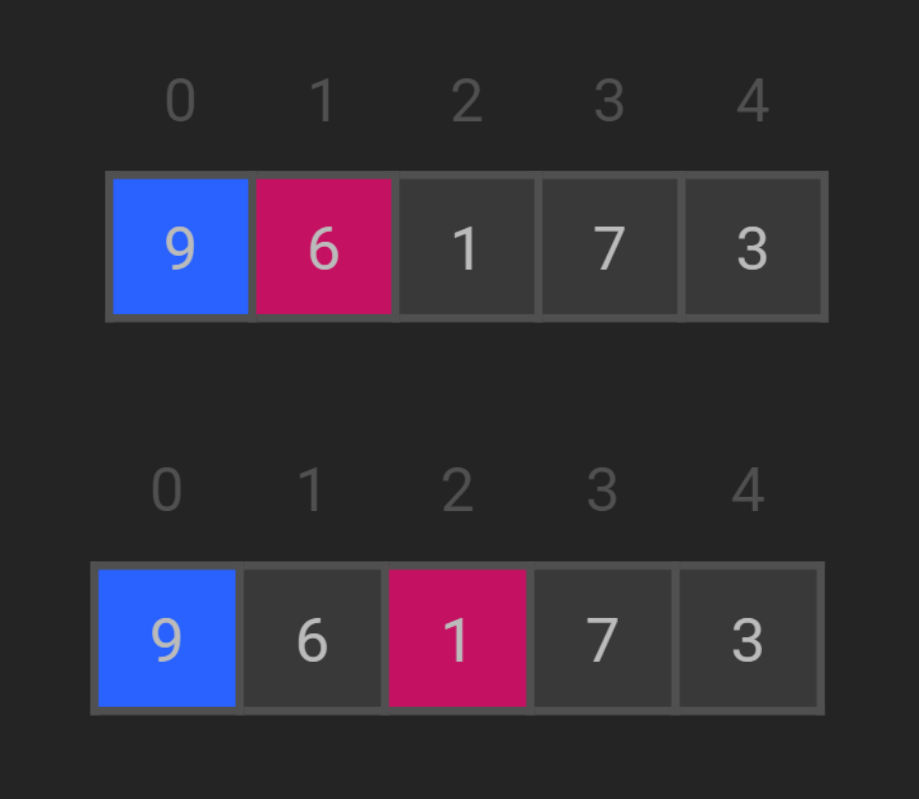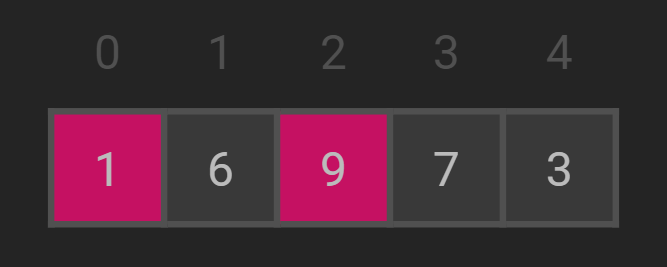Selection Sort

Data Structures SORTING ALGORITHMS SERIESIntroduction

Selection Sort is an iterative and in-place sorting algorithm that divides the data structure into two sublists: the ordered one, and the unordered one. The algorithm loops for all the elements of the data structure and for every cycle picks the smallest element of the unordered sublist and adds it to the sorted sublist, progressively filling it.

How does Selection Sort work?

Suppose we have an array [9, 6, 1, 7, 3] that we are willing to sort in ascending order.

1. Select the first element as minimum.2. Compare minimum with the second element. If the second element is smaller than minimum, assign the second element as minimum.
Compare minimum with the third element. Again, if the third element is smaller, then assign minimum to the third element otherwise do nothing. The process goes on until the last element.3. After each iteration, minimum is placed in the front of the unsorted list.4. For each iteration, indexing starts from the first unsorted element. Step 1 to 3 are repeated until all the elements are placed at their correct positions.

Algorithm

selectionSort(array, size)
repeat (size - 1) times
set the first unsorted element as the minimum
for each of the unsorted elements
if element < currentMinimum
set element as new minimum
swap minimum with first unsorted position
end selectionSort

Code Implementation

In Java

package algorithms.sorting;

import java.util.Arrays;

public class SelectionSort {
public static void main(String[] args) {
int[] nums = {9, 6, 1, 7, 3};
SelectionSort ss = new SelectionSort();
ss.sort(nums);
System.out.println(Arrays.toString(nums));
}

public void sort(int[] arr) {
for (int i = 0; i < arr.length - 1; i++) {
int minimum = i;
for (int j = i + 1; j < arr.length; j++) {
if (arr[j] < arr[minimum]) {
minimum = j;
}
}

// swap
int temp = arr[i];
arr[i] = arr[minimum];
arr[minimum] = temp;
}
}
}

In Python

def selection_sort(nums):
for i in range(len(nums)):
minimum = i
for j in range(i+1, len(nums)):
if nums[j] < nums[minimum]:
minimum = j

# Swap
nums[i], nums[minimum] = nums[minimum], nums[i]

if __name__ == "__main__":
data = [9, 6, 1, 7, 3]
selection_sort(data)
print(data)

Output

[1, 3, 6, 7, 9]

Selection Sort Complexity

Time Complexity Space Complexity Best O(n2) Worst O(n2) Average O(n2) O(1) No

Detailed Complexity

Cycle Number of Comparison
1st (n-1)
2nd (n-2)
3rd (n-3)
... ...
last 1

Number of comparisons: (n - 1) + (n - 2) + (n - 3) + ..... + 1 = n(n - 1) / 2 nearly equals to n2.

Complexity =  O(n2)

Time Complexity

• Worst Case Complexity: O(n2)
If we want to sort in ascending order and the array is in descending order then, the worst case occurs.
• Best Case Complexity: O(n2)
It occurs when the array is already sorted
• Average Case Complexity: O(n2)
It occurs when the elements of the array are in jumbled order (neither ascending nor descending).

Space Complexity

Space complexity is O(1) because an extra variable temp is used.

Conclusion

In this blog, we discussed the second sorting algorithm - Selection Sort. This blog post cannot end without thanking the person who has motivated a lot of students to learn DSA, that too free of cost - Kunal Kushwaha and Community Classroom. You can also access his free DSA playlist here.

Watch his Selection Sort video here :

Visualize algorithms easily on https://algorithm-visualizer.org/.